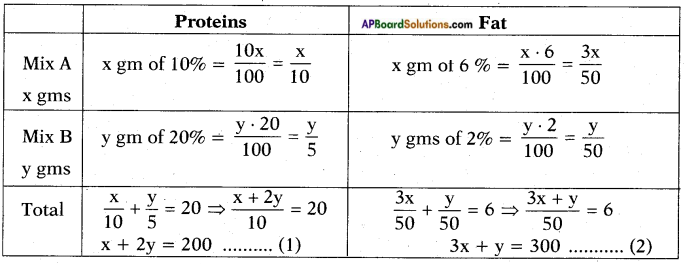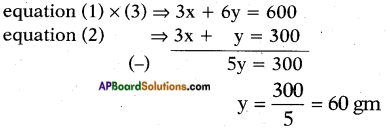# AP SSC 10th Class Maths Solutions Chapter 4 Pair of Linear Equations in Two Variables Optional Exercise

AP State Board Syllabus AP SSC 10th Class Maths Textbook Solutions Chapter 4 Pair of Linear Equations in Two Variables Optional Exercise Textbook Questions and Answers.

## AP State Syllabus SSC 10th Class Maths Solutions 4th Lesson Pair of Linear Equations in Two Variables Optional Exercise

### 10th Class Maths 4th Lesson Pair of Linear Equations in Two Variables Optional Exercise Textbook Questions and Answers

Question 1.
i) $$\frac{2x}{a}$$ + $$\frac{y}{b}$$ = 2
$$\frac{x}{a}$$ – $$\frac{y}{b}$$ = 4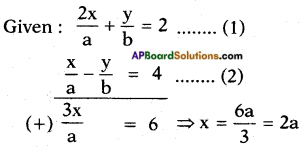Substituting x = 2a in the equation (1) we get
$$\frac{2}{a}$$(2a) + $$\frac{y}{b}$$ = 2
⇒ 4 + $$\frac{y}{b}$$ = 2
⇒ $$\frac{y}{b}$$ = -2
⇒ y = -2b
∴ The solution (x, y) = (2a, -2b)ii) $$\frac{x+1}{2}$$ + $$\frac{y-1}{3}$$ = 8
$$\frac{x-1}{3}$$ + $$\frac{y+1}{2}$$ = 9
Given: $$\frac{x+1}{2}$$ + $$\frac{y-1}{3}$$ = 8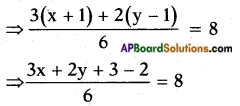⇒ 3x + 2y + 1 = 48
⇒ 3x + 2y = 47 …… (1)
and $$\frac{x-1}{3}$$ + $$\frac{y+1}{2}$$ = 9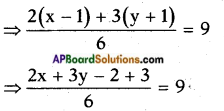⇒ 2x + 3y + 1 = 54
⇒ 2x + 3y = 53 …… (2)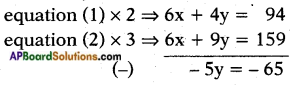⇒ y = $$\frac{-65}{-5}$$ = 13
Substituting y = 13 in equation (1) we get
3x + 2(13) = 47
⇒ 3x = 47 – 26
⇒ 3x = 21
⇒ x = $$\frac{21}{3}$$ = 7
∴ The solution (x, y) = (7, 13)

iii) $$\frac{x}{7}$$ + $$\frac{y}{3}$$ = 5
$$\frac{x}{2}$$ – $$\frac{y}{9}$$ = 6
Given: $$\frac{x}{7}$$ + $$\frac{y}{3}$$ = 5 and $$\frac{x}{2}$$ – $$\frac{y}{9}$$ = 6
⇒ $$\frac{3x+7y}{21}$$ = 5 and $$\frac{9x-2y}{18}$$ = 6
⇒ 3x + 7y = 105 …….. (1) and
9x – 2y = 108 …….. (2)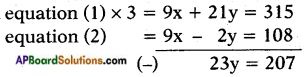⇒ y = $$\frac{207}{23}$$ = 9
Substituting y = 9 in equation (1) we get
3x + 7(9) = 105
⇒ 3x = 105 – 63
⇒ 3x = 42
⇒ x = $$\frac{42}{3}$$ = 14
∴ The solution (x, y) = (14, 9)iv) √3x + √2y = √3
√5x + √3y= √3
Given that √3x + √2y = √3 …… (1)
√5x + √3y = √3 …… (2)
By following elimination method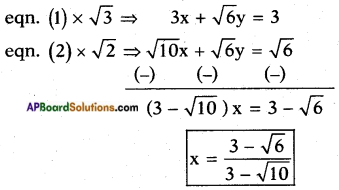Now again following elimination method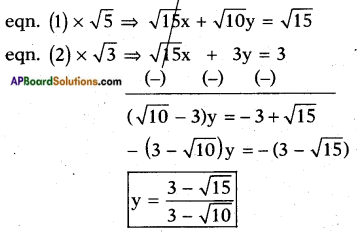∴ The solution x = $$\frac{3-\sqrt{6}}{3-\sqrt{10}}$$ and y = $$\frac{3-\sqrt{15}}{3-\sqrt{10}}$$

v) $$\frac{ax}{b}$$ + $$\frac{by}{a}$$ = a + b
ax – by = 2ab
Given: $$\frac{ax}{b}$$ + $$\frac{by}{a}$$ = a + b ……. (1)
ax – by = 2ab …….. (2)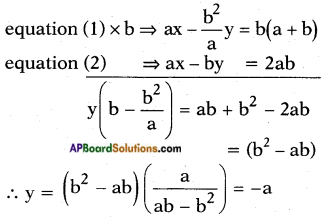Substituting y = – a in equation (2)
we get ax – b(-a) = 2ab
ax + ab = 2ab
ax = 2ab – ab = ab
⇒ x = $$\frac{ab}{a}$$ = b
∴ (x, y) = (b, -a)vi) 2x + 3y = 17
2x+2 – 3y+1 = 5
Given: 2x + 3y = 17 and
2x+2 – 3y+1 = 5
Take 2x = a and 3y = b then the given equations reduce to
2x + 3y = 17 ⇒ a + b = 17 …… (1)
2x . 22 – 3y . 3 = 5 ⇒ 4a – 3b = 5 …… (2)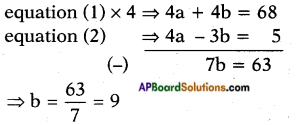Substituting b = 9 in equation (1) we get
a + 9 = 17 ⇒ a = 17 – 9 = 8
But a = 2x – 8 and b = 3y = 9
⇒ 2x = 23 and 3y = 32
⇒ x = 3 and y = 2
∴ The solution (x, y) is (3, 2)Question 2.
Animals in an experiment are to be kept on a strict diet. Each animal is to receive among other things 20g of protein and 6g of fat. The laboratory technicians purchased two food mixes, A and B. Mix A has 10% protein and 6% fat. Mix B has 20% protein and 2% fat. How many grams of each mix should be used?